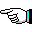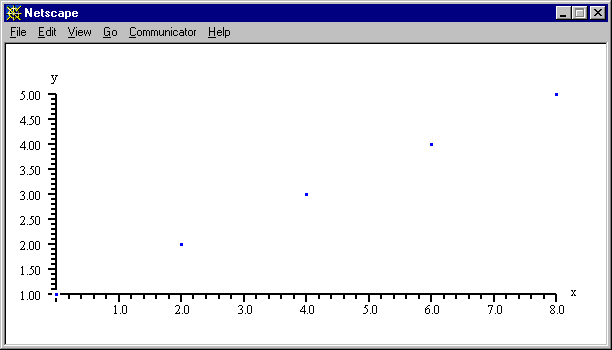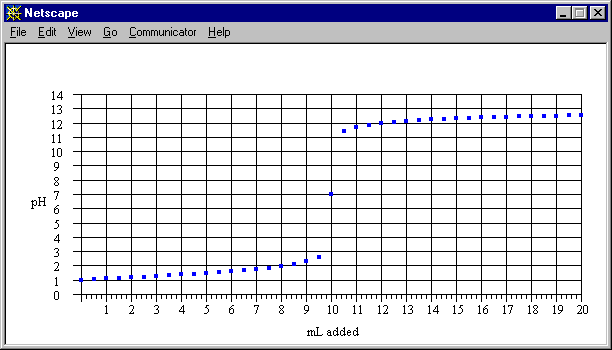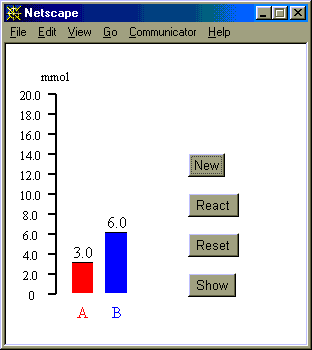Examples Involving a User Interface

There are several ways the user might be involved in creating a plot.

• In Example i1, the user is prompted for the function or x,y data to plot, because it is not provided.
• In Example i2, the function is specified, so the user is only prompted for the minimum and maximum x-value to use.
• Examples i3, i3a, and i4 illustrate how a user might introduce data using a textarea.
• Examples i5 and i6 demonstrate how feedback can be given to a user after a plot is drawn. This can be either automatic (as in Example i5) or upon the user pressing a link (as in Example i6).
• Example i7 demonstrates how divgraph.js can be used to create an animated histogram with button conrol.
• Example i8 demonstrates how divgraph.js can be used to create an "NMR spectrum" that is based on user data and is expandable to see finer detail.GRopengraphwindow(600,300) Info=new Array() Info.graphleft=Info.graphtop=50 Info.graphwidth=500 GRdrawgraph(document.examplei3.data.value,"","",Info) GRclosegraphdocument()GRopengraphwindow(600,300) Info=new Array() Info.graphleft=75 Info.graphtop=50 Info.graphwidth=500 Info.doptlabels=Info.dopoints=true Info.doxticnums=Info.doyticnums=true Info.dogridx=Info.dogridy=true Info.xaxislabel="mL added" Info.xaxislabelx=NaN Info.xaxislabely=NaN Info.yaxislabel="pH" Info.yaxislabelx=NaN Info.yaxislabely=NaN Info.xmin = 0 Info.xmax = NaN Info.ymin = 0 Info.ymax = 14 Info.defaultcolor="blue" Info.ptsize=2 Info.ticwidth=1 Info.xticfirst=0 Info.xticmajor=1 Info.xticminor=.2 Info.yticfirst=0 Info.yticmajor=1 Info.yticminor=1 Info.maxaddpoints=0 Info.ptsize=4 Info.docurve=false Info.dopoints=true Info.xnumdigits=Info.ynumdigits=0 GRdrawgraph(document.examplei3a.data.value,"","",Info) GRclosegraphdocument()
 GRopengraphwindow(500,300,50,200) Info=new Array() Info.graphleft=Info.graphtop=50 Info.ptsize=4 Info.linewidth=2 Info.docurve=document.examplei5.curve.checked Info.dotrendline=document.examplei5.trend.checked Info.style=".trendeqn {font-size:10pt}" Info.trendlabelx=250 Info.trendlabely=40 Info.xticmajor=Info.xticminor=Info.yticmajor=Info.yticminor=0 Info.doxticnums=Info.doyticnums=true Info.yvar="[NO2]" Info.xvar="time" Info.whendone=(Info.dotrendline?"check('i5')":"") fx=document.examplei5.horiz[document.examplei5.horiz.selectedIndex].value fy=document.examplei5.vert[document.examplei5.vert.selectedIndex].value Info.datatransform=fy +" vs. "+fx GRdrawgraph(sdata.replace(/ /g,","),"","",Info) GRclosegraphdocument()
 GRopengraphwindow(600,300,50,50) Info=new Array() Info.graphwidth=500,Info.graphheight=200 Info.graphleft=Info.graphtop=50 Info.ptsize=4 Info.linewidth=2 Info.docurve=document.examplei6.curve.checked Info.dotrendline=document.examplei6.trend.checked Info.trendlabelx=300 Info.trendlabely=-40 Info.doxticnums=Info.doyticnums=true Info.dogridx=Info.dogridy=true Info.xaxislabelx=Info.xaxislabely=NaN Info.ticwidth=1 Info.ptsize=document.examplei6.ptsize[document.examplei6.ptsize.selectedIndex].text Info.defaultcolor=document.examplei6.dcolor[document.examplei6.dcolor.selectedIndex].text Info.curvecolor=Info.trendcolor=document.examplei6.ccolor[document.examplei6.ccolor.selectedIndex].text Info.xvar=document.examplei6.xvar.value Info.yvar=document.examplei6.yvar.value fx=document.examplei6.horiz[document.examplei6.horiz.selectedIndex].text fy=document.examplei6.vert[document.examplei6.vert.selectedIndex].text Info.datatransform=fy +" vs. "+fx Info.title=(Info.dotrendline?"
Check":"")+"  Close" GRdrawgraph(document.examplei6.data.value,"","",Info) GRclosegraphdocument()GRopengraphwindow() Data=new Array([NaN,NaN]) Info=new Array() Info.maxaddpoints=12 Info.xmin=Info.ymin=0 Info.xmax=12 Info.ymax=20 Info.doxaxis=false Info.yticmajor=2 Info.yticminor=2 Info.xaxislabel="" Info.yaxislabel="mmol" GRdrawgraph("",Data,"",Info) GRclosegraphdocument() ptAbar=GRaddpoint(1,0) ptBbar=GRaddpoint(3,0) ptAtop=GRaddpoint(1,0) ptBtop=GRaddpoint(3,0) ptAn=GRaddpoint(1.1,-2) ptBn=GRaddpoint(3.1,-2) GRaddtext(1.3,-1,"A","red") GRaddtext(3.4,-1,"B","blue") GRaddtext(8,14,"back to the list of examples